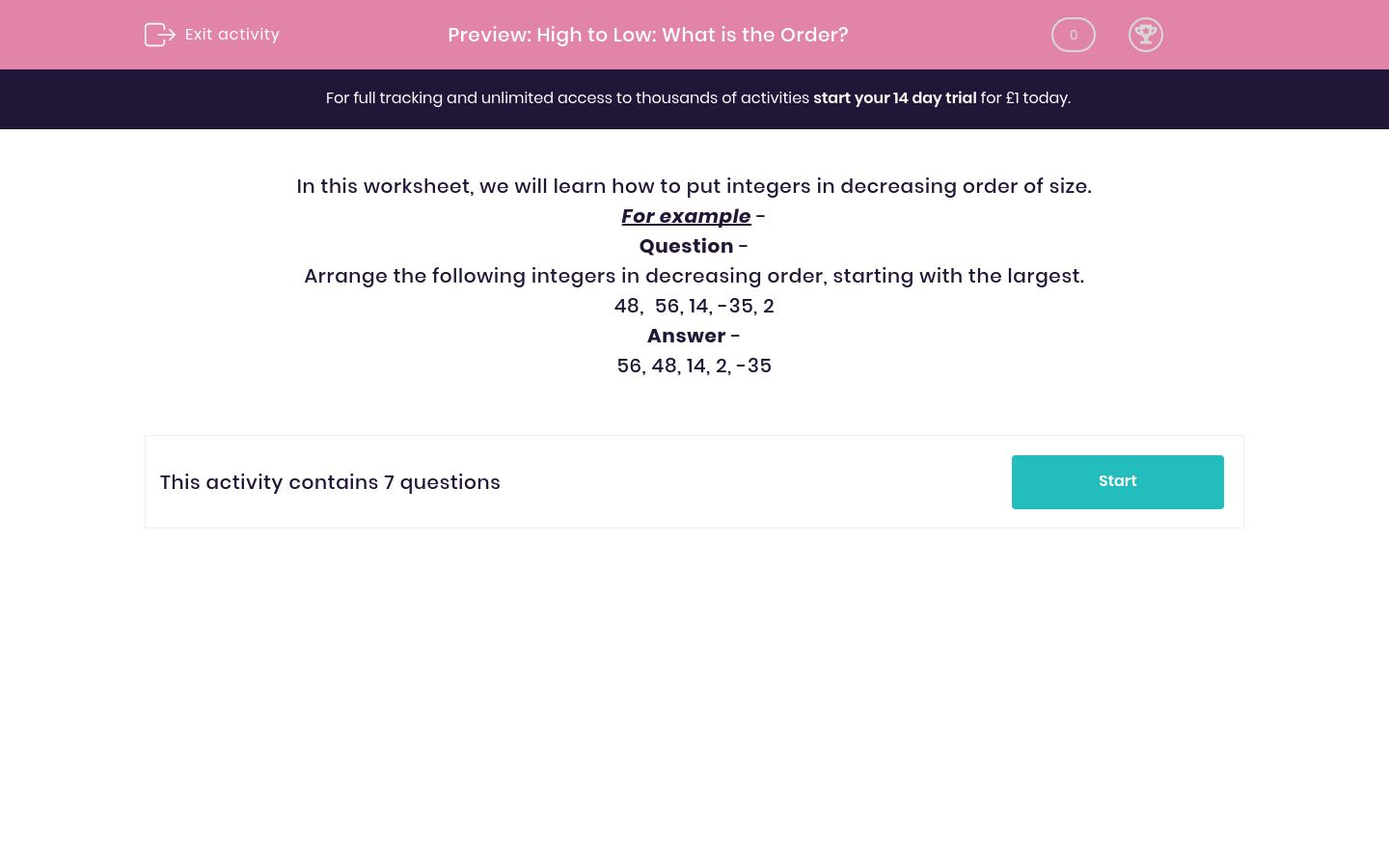# High to Low: What is the Order?

In this worksheet, students rearrange numbers in decreasing order of size.Key stage:  KS 2

Curriculum topic:   Maths and Numerical Reasoning

Curriculum subtopic:   Place Value

Difficulty level:### QUESTION 1 of 10

In this worksheet, we will learn how to put integers in decreasing order of size.

For example -

Question -

Arrange the following integers in decreasing order, starting with the largest.

48,  56, 14, -35, 2

56, 48, 14, 2, -35

Arrange the following integers in order, putting the largest first.

-47,  37, 16, -26, -5

Arrange the following integers in order, starting with the largest.

-17,  -41, -29, -35, -23

Arrange the following integers in decreasing order.

-22, -49, -40, -13, -31

Arrange the following integers in decreasing order.

19, -15, -49, 2, -32

Arrange the following integers in order, starting with the largest.

-14, -46, -30, 18, 2

Arrange the following integers in order, starting with the largest.

-41, -44, -35, -47, -38

Arrange the following integers in decreasing order.

-2, -41, 11, -28, -15

• Question 1

Arrange the following integers in order, putting the largest first.

-47,  37, 16, -26, -5

37
16
-5
-26
-47
• Question 2

Arrange the following integers in order, starting with the largest.

-17,  -41, -29, -35, -23

-17
-23
-29
-35
-41
EDDIE SAYS
-17 is the largest number here.
• Question 3

Arrange the following integers in decreasing order.

-22, -49, -40, -13, -31

-13
-22
-31
-40
-49
• Question 4

Arrange the following integers in decreasing order.

19, -15, -49, 2, -32

19
2
-15
-32
-49
• Question 5

Arrange the following integers in order, starting with the largest.

-14, -46, -30, 18, 2

18
2
-14
-30
-46
• Question 6

Arrange the following integers in order, starting with the largest.

-41, -44, -35, -47, -38

-35
-38
-41
-44
-47
• Question 7

Arrange the following integers in decreasing order.

-2, -41, 11, -28, -15

11
-2
-15
-28
-41
---- OR ----